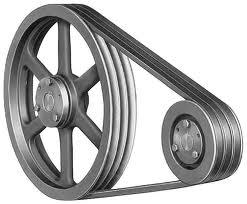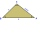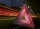# V-belt

Calculate the length of the belt on pulleys with diameters of 105 mm and 393 mm at shaft distance 697 mm.

Result

l =  2.2 m

#### Solution:

$\ \\ r_1 = d_1/2 = 52.5 \ mm \ \\ r_2 = d_2/2 = 196.5 \ mm \ \\ \Delta r = r_2-r_1 = 144 \ mm \ \\ \alpha = arctg(\dfrac{\Delta r} {l}) = arctg(\dfrac{ 144 } { 697 }) = 11.67^\circ = 0.203733309106 \ \\ dr = \sqrt{ l^2-(\Delta r)^2 } = \sqrt{ 697 ^ 2-( 144 )^2 } = 681.96 \ mm \ \\ l_1 = r_1\cdot (\pi-2\cdot \alpha) = 143.54 \ mm \ \\ l_2 = r_2\cdot (\pi+2\cdot \alpha) = 697.39 \ mm \ \\ l = l_1+l_2 + 2 \cdot dr = \dfrac{ 143.54+697.39+2 \cdot 681.96 }{1000} = 2.2 \ \text{m} \ \\$Our examples were largely sent or created by pupils and students themselves. Therefore, we would be pleased if you could send us any errors you found, spelling mistakes, or rephasing the example. Thank you!

Leave us a comment of this math problem and its solution (i.e. if it is still somewhat unclear...):Be the first to comment!Tips to related online calculators
Pythagorean theorem is the base for the right triangle calculator.

#### You need to know the following knowledge to solve this word math problem:

We encourage you to watch this tutorial video on this math problem:

## Next similar math problems:

1. The right triangleThe right triangle ABC has a leg a = 36 cm and an area S = 540 cm2. Calculate the length of the leg b and the median t2 to side b.
2. The trianglesThe triangles KLM and ABC are given, which are similar to each other. Calculate the lengths of the remaining sides of the triangle KLM, if the lengths of the sides are a = 7 b = 5.6 c = 4.9 k = 5
3. Lookout towerCalculate the height of a lookout tower forming a shadow of 36 m if at the same time a column 2.5 m high has a shadow of 1.5 m.
4. The basesThe bases of the isosceles trapezoid ABCD have lengths of 10 cm and 6 cm. Its arms form an angle α = 50˚ with a longer base. Calculate the circumference and content of the ABCD trapezoid.
5. In aIn a triangle, the aspect ratio a: c is 3: 2 and a: b 5: 4. The perimeter of the triangle is 74cm. Calculate the lengths of the individual sides.
6. Annulus from triangleCalculate the content of the area bounded by a circle circumscribed and a circle inscribed by a triangle with sides a = 25mm, b = 29mm, c = 36mm
7. Triangular prismCalculate the surface of a regular triangular prism, the edges of the base are 6 cm long and the height of the prism is 15 cm.
8. Interior anglesCalculate the interior angles of a triangle that are in the ratio 2: 3: 4.
9. Spherical capThe spherical cap has a base radius of 8 cm and a height of 5 cm. Calculate the radius of a sphere of which this spherical cap is cut.
10. Concentric circles and chordIn a circle with a diameter d = 10 cm, a chord with a length of 6 cm is constructed. What radius have the concentric circle while touch this chord?
11. Observation towerFrom the observation tower at a height of 105 m above sea level, the ship is aimed at a depth angle of 1° 49´. How far is the ship from the base of the tower?
12. Cone roofHow many m2 of roofing is needed to cover a cone-shaped roof with a diameter of 10 m and a height of 4 m? Add an extra 4% to the overlays.
13. The conicalThe conical candle has a base diameter of 20 cm and a side of 30 cm. How much dm ^ 3 of wax was needed to make it?
14. The anglesThe angles in the triangle are in the ratio 12: 15: 9. Find the angles.
15. ProbabilityHow probable is a randomly selected three-digit number divisible by five or seven?
16. FlakesA circle was described on the square, and a semicircle above each side of the square was described. This created 4 "flakes". Which is bigger: the content of the central square or the content of four chips?
17. The angles ratioThe angles in the ABC triangle are in the ratio 1: 2: 3. find the sizes of the angles and determine what kind of a triangle it is.